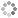# PROWAREtecharticles » current » javascript » tutorial » page-05

## JavaScript: Tutorial - A Guide to JavaScript - Page 05

Language Basics (Operators: Bitwise, Boolean, Additive).

### Bitwise Operators

The bitwise operators are almost the same as they are in the C/C++ language.

 `~` Bitwise NOT operator `&` Bitwise AND operator `^` Bitwise XOR operator `|` Bitwise OR operator `<<` Bitwise LEFT SHIFT operator `>>` Bitwise SIGNED RIGHT SHIFT operator `>>>` Bitwise UNSIGNED RIGHT SHIFT operator

### Boolean Operators

#### Logical NOT

The logical NOT operator is an exclamation point (`!`). It first converts the operand to a Boolean value and then negates it. `true` is returned if the operand is undefined. `true` is returned if the operand is NaN. `true` is returned if the operand is null. `false` is returned if the operand is any number other than 0 (including Infinity). `true` is returned if the operand is the number 0. `false` is returned if the operand is a non-empty string. `true` is returned if the operand is an empty string. `false` is returned if the operand is an object.

One can simulate the behavior of the Boolean() casting function by using two NOT operators in a row.

``````alert(!!0);	 // false

#### Logical AND

The logical AND operator is a double ampersand (`&&`).

``````if(true && true) {
}``````

Logical AND can be used with any type of operand, not just Boolean values. When any operand is not a primitive Boolean, logical AND does not always return a Boolean value. If the first operand is an object, the second operand is always returned. If the second operand is an object, the object is returned only if the first operand evaluates to `true`. If both operands are objects, the second operand is returned. If either operand is `null`, `null` is returned. If either operand is `NaN`, `NaN` is returned. If either operand is `undefined`, `undefined` is returned. If the first operand determines the result, the second operand is never evaluated. So, if the first operand is `false`, no matter what the value of the second operand, the expression can not be `true`.

#### Logical OR

The logical OR operator is a double pipe (`||`).

``````if(true || false) {
}``````

Just like logical AND, if any operand is not a Boolean, logical OR will not always return a Boolean value. If the first operand is an object, the first operand is returned. If the first operand evaluates to `false`, the second operand is returned. If both operands are objects, the first operand is returned. If both operands are `null`, `null` is returned. If both operands are `NaN`, `NaN` is returned. If both operands are `undefined`, `undefined` is returned. if the first operand evaluates to `true`, the second operand is not evaluated. So, if the first operand is `true`, no matter what the value of the second operand, the expression can not be `false`.

This behavior can be used to avoid assigning a null or undefined value to a variable. Consider this:

``var obj = preferredObject || backupObject;``

The variable `obj` will be assigned one of two values. The `preferredObject` variable contains the value that is preferred if it is available, whereas the `backupObject` variable contains the backup value if the preferred one isn't available. If `preferredObject` is not null, then it is assigned to `obj`; if it is `null`, then `backupObject` is assigned to `obj`.

The add operator is a plus sign (`+`).

``````var sum = 3 + 5;

If the two operands are numbers then an add is performed. If either operand is `NaN` then the result is `NaN`. If `Infinity` is added to `Infinity` then the result is `Infinity`. If `–Infinity` is added to `–Infinity` then the result is `–Infinity`. If `Infinity` is added to `–Infinity` then the result is `NaN`. If +0 is added to +0 then the result is +0. If –0 is added to +0 then the result is +0. If –0 is added to –0 then the result is –0.

If one of the operands is a string then they are concatenated. If both operands are strings then the second string is concatenated to the first. If only one operand is a string then the other operand is converted to a string and the result is the concatenation of the two strings.

If either operand is an object, number, or Boolean, its `toString()` method is called to get a string value and then the previous rules regarding strings are applied. The `String()` function is called to retrieve the values `"undefined"` and `"null"` for `undefined` and `null`. This code demonstrates this:

``````var num1 = 5;
var num2 = 3;
var message = "The sum of 5 and 3 is " + num1 + num2;
alert(message); // "The sum of 5 and 3 is 53"``````
``````var num1 = 5;
var num2 = 3;
var message = "The sum of 5 and 3 is " + (num1 + num2);
alert(message); // "The sum of 5 and 3 is 8" (THIS IS THE DESIRED RESULT)``````
[Page 5 of 22]This site uses cookies. Cookies are simple text files stored on the user's computer. They are used for adding features and security to this site. Read the privacy policy.